# High School Physics : Understanding Effects of Heat on Volume

## Example Questions

### Example Question #21 : Thermodynamics

An ideal gas is inside of a container with a pressure of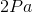. If it starts with a volume of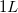and is compressed to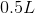, what is the new pressure if the temperature remains constant?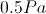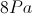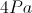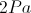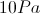Explanation:

We will need to use Boyle's Law to solve: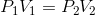Boyle's Law allows us to set up a relationship between the changes in pressure and volume under conditions with constant temperature. Since the equation is a proportion, we do not need to convert any units.

We can use the given values to solve for the new pressure.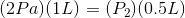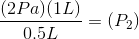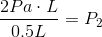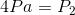### Example Question #22 : Thermodynamics

An ideal gas is inside of a tube at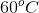. If the pressure remains constant, but the volume decreases from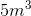to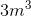, what will be the final temperature in the tube?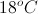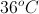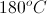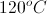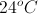Explanation:

For this problem, use Charles's Law: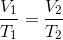In this formula,is the volume and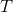is the temperature. Charles's Law allows us to set up a proportion for changes in volume and temperature, as long as pressure remains constant. Since we are dealing with a proportion, the units for temperature are irrelevant and we do not need to convert to Kelvin.

Using the given values, we should be able to solve for the final temperature.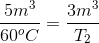Cross multiply.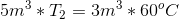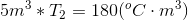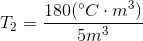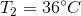### Example Question #1 : Understanding Effects Of Heat On Volume

An ideal gas is compressed from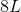to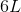at constant temperature. If the initial pressure was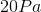, what is the new pressure?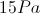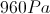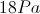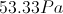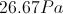Explanation:

For this problem, use Boyle's Law:Boyle's Law allows us to set up a proportion between the pressure and volume at a constant temperature.

Using the values given, we can solve for the final pressure.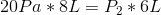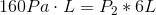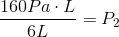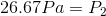### Example Question #1 : Understanding Effects Of Heat On Volume

A balloon in a hot room is submerged in a bucket of cold water. What will happen to this balloon?

It will lose air

It will expand

It will pop

It will not change

It will shrink

It will shrink

Explanation:

The volume of air in the balloon will increase when exposed to hotter temperatures, and decrease when exposed to colder temperatures. If we look at the ideal gas law, we can see that temperature and volume have a direct relationship. As one goes down, so does the other, assuming all other factors remain constant.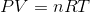We can also look at Charles's law of volumes:The balloon is sealed, so the amount of gas in the balloon will not change, and the elasticity of the balloon means that pressure will also remain constant. As temperature decreases, volume must also decrease. Suppose that the temperature is halved in our question. The result would be half the volume, according to Charles's law.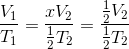By this logic, we can conclude that the balloon will shrink when placed in the cold water.

### Example Question #31 : Thermodynamics

Why does adding heat cause a gas to expand?

Adding heat increases the velocity of the molecules

Adding heat will not cause a gas to expand

Adding heat causes the molecules to bond together, increasing their volume

Adding heat reduces the friction in the molecules when they are moving